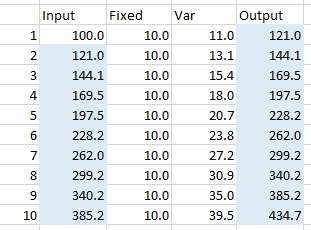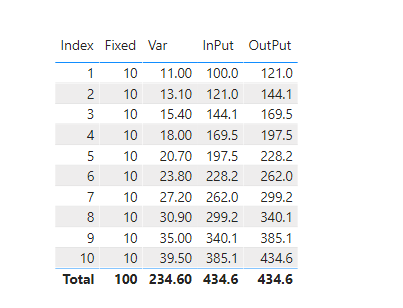cancel
Showing results for
Did you mean:Anonymous
Not applicable

## Theoretical formula to help build amortisation table

Suppose I have an input number of 100.

For each period I have a fixed number which I will add to the latest input number and then I will multiply it by 0.1 and add it to the total (or multiple by 1.1).

i.e.

Round 1:

Input = 100 + 10 (fixed number) = 110
110 * 1.1 = 121 (new input)

Round 2:

Input = 121 + 10 (fixed number) = 131
131 * 1.1 = 144.1

etc.

I need a way to write a calculated measure or calcualted table to work out the variable amount and closing amount at any given round. Chart is below.Would appreciate anyone who thinks they're able to write a formula for this. My trouble is that the variable column is a self-reference, since to calculate the variable number in a certain period, you need to know the variable number in all previous periods to work out the input number for the period you are trying to calculate.

Thanks!

1 ACCEPTED SOLUTIONCommunity Support

Hi @Anonymous ,

Try the following measures

``````InPut =
VAR _Firstinput = 100
VAR _Fixedbefore = CALCULATE(SUM('Table'[Fixed]),FILTER(ALL('Table'),'Table'[Index]<SUM('Table'[Index])))
VAR _Varbefore = CALCULATE(SUM('Table'[Var]),FILTER(ALL('Table'),'Table'[Index]<SUM('Table'[Index])))
RETURN
_Firstinput+_Fixedbefore+_Varbefore``````
``````OutPut =
VAR _Firstinput = 100
VAR _Fixedbefore = CALCULATE(SUM('Table'[Fixed]),FILTER(ALL('Table'),'Table'[Index]<=SUM('Table'[Index])))
VAR _Varbefore = CALCULATE(SUM('Table'[Var]),FILTER(ALL('Table'),'Table'[Index]<=SUM('Table'[Index])))
RETURN
_Firstinput+_Fixedbefore+_Varbefore``````Best Regards,

Stephen Tao

If this post helps, then please consider Accept it as the solution to help the other members find it more quickly.

2 REPLIES 2Community Support

Hi @Anonymous ,

Try the following measures

``````InPut =
VAR _Firstinput = 100
VAR _Fixedbefore = CALCULATE(SUM('Table'[Fixed]),FILTER(ALL('Table'),'Table'[Index]<SUM('Table'[Index])))
VAR _Varbefore = CALCULATE(SUM('Table'[Var]),FILTER(ALL('Table'),'Table'[Index]<SUM('Table'[Index])))
RETURN
_Firstinput+_Fixedbefore+_Varbefore``````
``````OutPut =
VAR _Firstinput = 100
VAR _Fixedbefore = CALCULATE(SUM('Table'[Fixed]),FILTER(ALL('Table'),'Table'[Index]<=SUM('Table'[Index])))
VAR _Varbefore = CALCULATE(SUM('Table'[Var]),FILTER(ALL('Table'),'Table'[Index]<=SUM('Table'[Index])))
RETURN
_Firstinput+_Fixedbefore+_Varbefore``````Best Regards,

Stephen Tao

If this post helps, then please consider Accept it as the solution to help the other members find it more quickly.Anonymous
Not applicable

Another thing to add on is that the "fixed" addition may not be consistent throughout the entire periods so the formula needs to handle that as well.